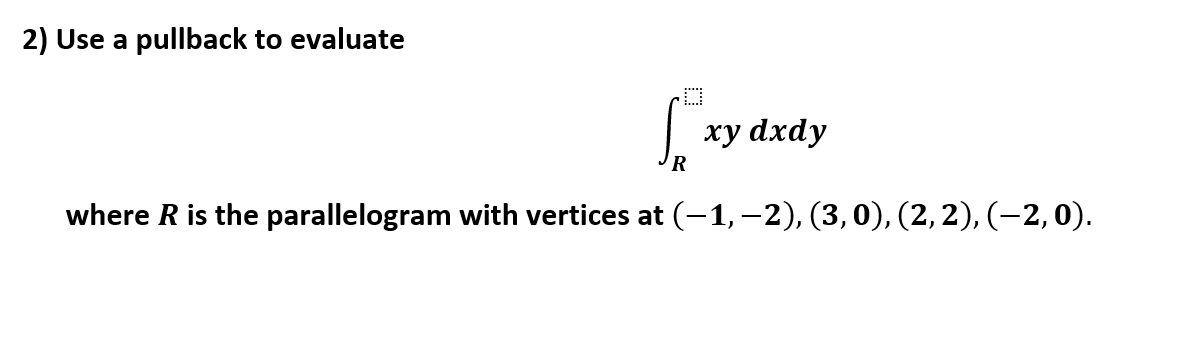# 2) Use a pullback to evaluatexy dxdyRwhere R is the parallelogram with vertices at (-1,-2), (3, 0), (2,2), (-2, 0)

Question
1 views

can u help me with pullbacks and integration plzhelp_outlineImage Transcriptionclose2) Use a pullback to evaluate xy dxdy R where R is the parallelogram with vertices at (-1,-2), (3, 0), (2,2), (-2, 0) fullscreen
check_circle

star
star
star
star
star
1 Rating
Step 1

Given that the integral,

Step 2

here, R is the parallelogram with vertices at (-1, -2), (3, 0), (2, 2), and (-2, 0).

Equation of line through the point (-1, -2), (3, 0) is

x - 2y = 3

Equation of line through the point (3, 0), (2, 2) is

2x + y = 6

Equation of line through the point (2, 2), (-2, 0) is

x - 2y = - 2

Equation of line through the point (-2, 0), (-1, -2) is

2x + y = - 4

Assume that,

x - 2y = u

and

2x + y = v

Then, u = 3 and u = - 2

v = 6 and v = - 4

Also,  x - 2y = u

2x + y = v

This implies that,

Step 3

Use the pullback (method of change of variable), for this find the value of Jacobian ...

### Want to see the full answer?

See Solution

#### Want to see this answer and more?

Solutions are written by subject experts who are available 24/7. Questions are typically answered within 1 hour.*

See Solution
*Response times may vary by subject and question.
Tagged in

### Math